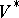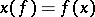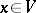Linear algebra

The branch of algebra in which one studies vector (linear) spaces, linear operators (linear mappings), and linear, bilinear and quadratic functions (functionals and forms) on vector spaces.

Historically the first branch of linear algebra was the theory of linear algebraic equations (cf. Linear equation). In connection with the solution of systems of linear equations there arose the concept of a determinant. In 1750 Cramer's rule (cf. Cramer rule) was obtained for the solution of a system of linear equations in which the number of equations is equal to the number of unknowns and in which the determinant of the coefficients at the unknowns is non-zero. In 1849 the Gauss method for solving systems of linear equations with numerical coefficients was proposed. This method is the simplest with respect to the number of operations applied and is also used, with various changes, for the approximate solution of systems of equations whose coefficients are also known approximately.

The concept of a matrix arose in connection with the study of systems of linear equations and their determinants. The concept of the rank of a matrix, proposed by G. Frobenius in 1877, made it possible to express explicitly the conditions for compatibility and determinacy of a system of linear equations in terms of the coefficients of the system (see Kronecker–Capelli theorem). The construction of the general theory of systems of linear equations was thus completed at the end of the 19th century.

If in the 18th century and 19th century the main content of linear algebra comprised systems of linear equations and the theory of determinants, then in the 20th century the central position was taken by the concept of a vector space and the associated concepts of a linear transformation, and a linear, bilinear and multilinear function on a vector space.

A vector, or linear, space over a fieldis a setof elements (called vectors) in which the operations of addition of vectors and multiplication of a vector by elements ofare specified and satisfy a number of axioms (see Vector space). Vector spaces over skew-fields are also considered. One of the most important concepts in the theory of vector spaces is that of a linear mapping, that is, a homomorphism of vector spaces over the same field. A linear operator, or linear transformation, is a linear mapping of the space into itself (that is, an endomorphism of the vector space). If the spaceis finite dimensional, then by choosing a basisinand puttingone obtains a square matrixof order, which is called the matrix of the linear transformationin the given basis.

A vector spaceover a fieldendowed with an additional operation of multiplication of vectors satisfying certain axioms, is called an algebra over(see Rings and algebras; Operator ring).

All linear transformations of a spacewith respect to the naturally defined operations of addition, multiplication and multiplication of linear transformations by elements ofform an algebra over. All square matrices of fixed order with elements fromalso form an algebra over. The correspondence mentioned above between linear transformations of a spaceand their matrices in a given basis is an isomorphism of these algebras, which makes it possible to formulate theorems about linear transformations in the language of matrices and to use the theory of matrices in proving them.

Of great significance in the theory of linear transformations is the choice of bases in which the matrix of a transformation takes its simplest form in some sense. In the case of an algebraically closed field this form is, for example, the Jordan normal form of the matrix.

An important case of a linear mapping is a linear function (linear functional) — a linear mapping ofinto. All linear functions onwith respect to the naturally defined operations of addition and multiplication by elements ofform a vector spaceover, called the space dual to. The vectors ofcan in turn be regarded as linear functions on the dual spaceby puttingfor all,. Ifis finite dimensional, this establishes a natural isomorphism betweenand.

A generalization of the concept of a linear function is the concept of a multilinear function, that is, a function with values in, depending on several arguments (of which some belong toand the others to), which is linear in each argument. These functions are also called tensors. Multilinear algebra is taken up with the study of them. A special case of multilinear functions are bilinear functions (see Bilinear mapping). Skew-symmetric multilinear functions are also called exterior forms (cf. Exterior form).

On the basis of the concept of a vector space various classical spaces studied in geometry have been defined: affine spaces, projective spaces, etc.

The theory of vector spaces has important connections with group theory. All automorphisms of an-dimensional vector spaceover a fieldform a group with respect to multiplication, isomorphic to the group of non-singular square matrices of orderwith elements from. A homomorphic mapping of some groupinto this group of automorphisms is called a linear representation of the groupin. The study of properties of representations is the subject of the theory of linear representations of groups (cf. also Linear representation).

The classical theory of linear equations and determinants has been generalized to the case when instead of numbers or elements of a field one considers elements of an arbitrary skew-field.

A natural generalization of the concept of a vector space over a fieldis the concept of a module over an arbitrary ring. Some basic theorems of linear algebra cease being true if the vector space is replaced by a module. The study of the possibility of generalizations that are also valid for modules led to the creation of algebraic-theory.